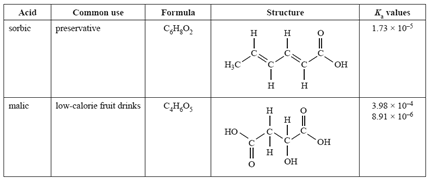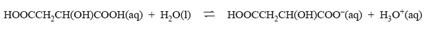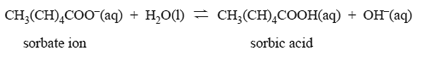Acid/base Equilibria (2012 VCE) 1) The weak acids shown in the table below are used in the food industry.a) What does the term 'weak acid' mean? Solutionb) Why are two Ka values listed for malic acid? Solution c) The equation related to the first Ka value of malic acid isWrite an appropriate chemical equation that relates to the second Ka of malic acid. Solutiond) Sorbic acid, CH3(CH)4COOH, has antimicrobial properties that are used to inhibit yeast and mould growth. However, its solubility is very low. The more soluble potassium sorbate is used instead. The antimicrobial activity of sorbic acid is retained because an equilibrium exists according to the equation below.How would the addition of a small amount of 1.0 M hydrochloric acid affect the concentration of sorbic acid in solution? Justify your answer in terms of equilibrium principles. Solutione) Calculate the percentage dissociation of sorbic acid when the pH = 4.76. Solution